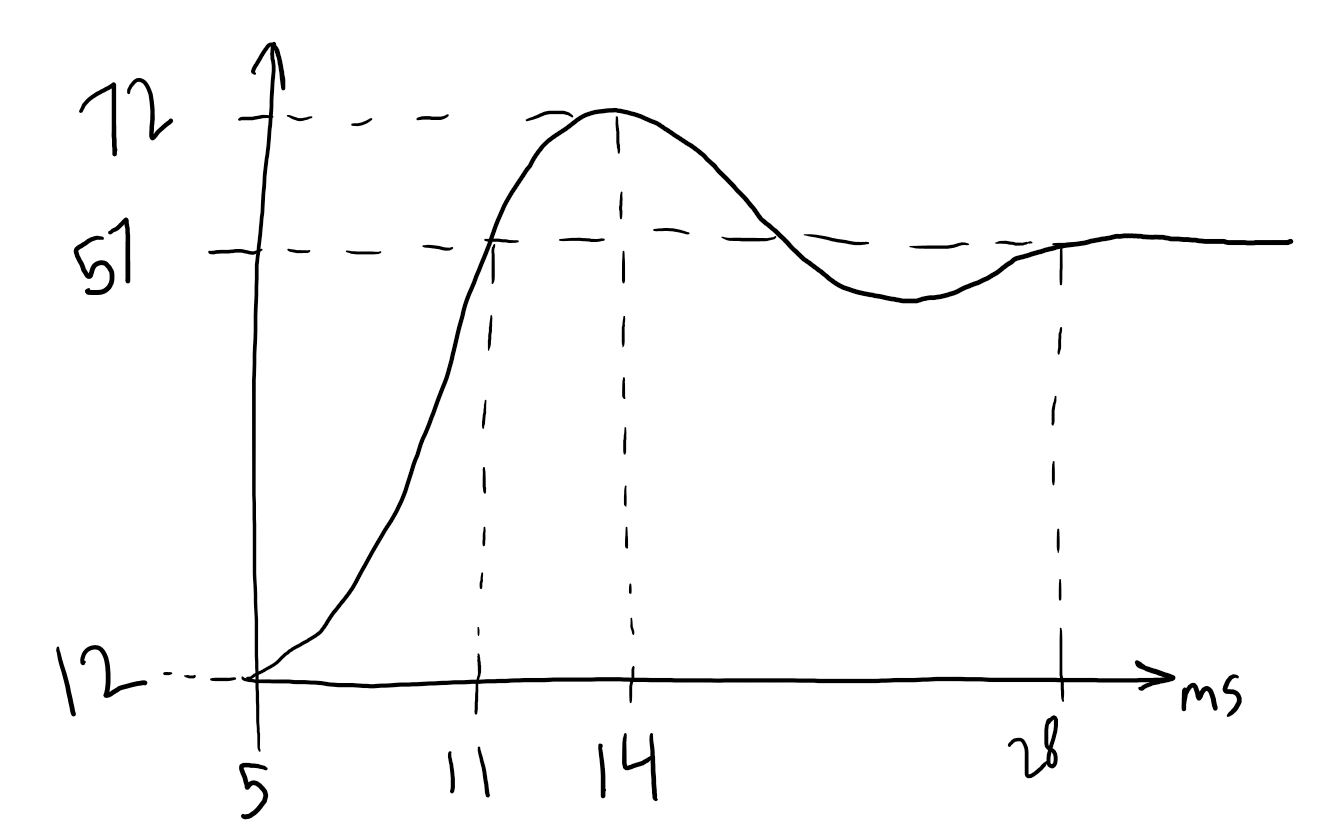Robotics 2
Checkpoint 3: ControlExample Question 2:  Suppose you are designing an application with a motor.  How might you decide between using a stepper motor, a DC motor, and a servo motor?  Give at least one example of a situation when you would choose each option.
Example Question 3:  Suppose that you are using PID control to position your robot joints.  You observe the step response, then you increase the proportional, integral, and derivative gains one at a time.  What would you expect to be the effects on overshoot, rise time, and steady-state error for each individual change?
Example Question 4:  Suppose that you are using proportional control alone, and you observe that the step response has too much overshoot and isn't accurate enough (there is too much steady-state error).  What change(s) to the controller would you try to fix these problems?
Example Question 5: Suppose you have captured a step response that looks like this.  Find values for the damping ratio, zeta, and the natural frequency, wn.
Example Question 7: For the system in the last problem, draw what the Bode plot would look like.
Example Question 8:  Suppose you are going to use the frequency response method to model a second-order system.  Explain what experiment you would do and how you would find the system model from your measurements.
Example Question 1: Suppose you are selecting a stepper motor for an application where your stepper motor is attached to a belt drive, and you know that the belt moves 20mm in one revolution of the motor.  You want to be able to position the thing attached to the belt with a precision of 0.1mm.  What is the minimum value of degrees per step that the motor needs to have?  If you then decide to move the belt at a speed of 10 mm/s, how long of a delay, in milliseconds, should you have between steps?
Example Question 9:  Suppose the step response in question 5 was captured while the system was under proportional control and Kp was set to 1.  Find the value of Kp that would make the system critically-damped.  Do you expect the correct answer to this question to be greater than or less than 1?  Say why you think that.
Example Question 10:  Now, suppose the step response in question 5 was captured while the system was under proportional control and Kp was set to 2.  Find the value of Kp that would give a zeta value of 0.7.
Congratulations!  You've made it all the way through our third unit: Control.  Before we move on, you will need to take a test to make sure that all this information has sunk in.  Here are some example problems like the ones you will see on the test in class.  On our review day (the class day before the test), I'll go through these problems live on the board.  So, it's a good idea to try to solve the problems on your own before coming to class.Example Question 6:  For the values you found in the last problem, what is the differential equation model of the system, and what is the transfer function?